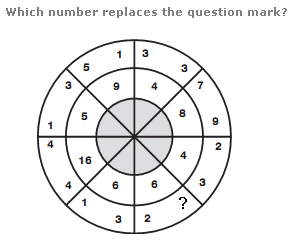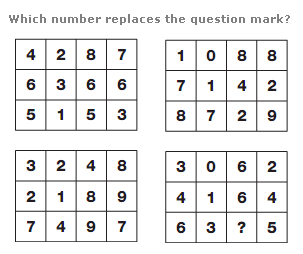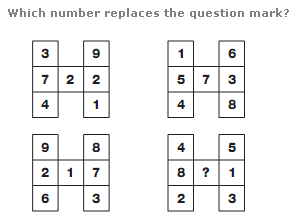# Puzzles - Number puzzles

### Exercise :: Number puzzlesAnswer : 7 Explanation : The inner digit in each segment equals the sum of the two numbers in the outer part of the opposite segment.Answer : 0 Explanation : In each row of each grid, multiply the left and right hand numbers together to give a digit value, and write this value in the two centre boxes.Answer : 5 Explanation : In each H shape, the central number is equal to the difference between the sum of the 3 left hand numbers and the sum of the 3 right hand numbers.